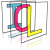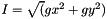Image Component Library (ICL)

Class for calculation of gradient image information. More...

#include <GradientImage.h>

## Public Types

enum  calculationMode { calculateXYOnly =0, calculateIntensity =1, calculateAngle =2, calculateAll =3 }
flags to indicate which gradient information should be calculated More...

## Public Member Functions

Empty constructor. More...

void update (const core::ImgBase *src, calculationMode mode=calculateAll)
Main function calculates new gradient image information. More...

void normalize ()
internally normalizes all images to range [0,255] More...

## Private Attributes

core::Img16s m_oBuf

core::Img16s m_oX

core::Img16s m_oY

core::Img32f m_oI

core::Img32f m_oA

## Detailed Description

Class for calculation of gradient image information.

The GradientImage class provides functionalities for the calculation of the following gradient image information:

• X-Gradient (using 3x3 Sobel-X Convolution Kenrnel)
• Y-Gradient (using 3x3 Sobel-Y Convolution Kenrnel)
• Gradient Intensity (internally accelerated using a LUT)• Gradient Angle (also accelerated using a LUT)For best performance, the input image is restricted to be of type Img8u. The update function will firstly calculate X- and Y-Gradient information using a ConvolutionOp object. X- and Y-Gradient image have range [0,255] now (result of Sobel-Filter is internally scaled to the range of the used datatype icl8u. Further calculation of gradient intensity and gradient angle depends on the given calculation mode flag.

## ◆ calculationMode

flags to indicate which gradient information should be calculated

Enumerator
calculateXYOnly

calculateIntensity

calculateAngle

calculateAll

## Constructor & Destructor Documentation

inline

Empty constructor.

inline

inline

inline

inline

## ◆ normalize()

internally normalizes all images to range [0,255]

## ◆ update()

 void icl::filter::GradientImage::update ( const core::ImgBase * src, calculationMode mode = calculateAll )

Main function calculates new gradient image information.

private

private